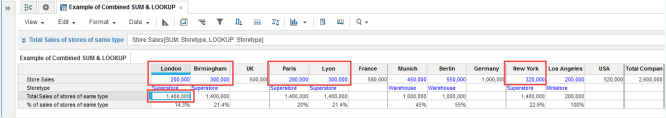1. Calculation functions
2. All Functions
3. Compound Functions
4. SUM and LOOKUPFirst it sums based on a list-formatted line item or property in the source, then looks up a value from a source module using one or more mappings.

## Syntax

This example of acceptable syntax uses two SUM mappings and three LOOKUP mappings:

`source[SUM: sum mapping 1, SUM: sum mapping 2, LOOKUP: lookup mapping 1, LOOKUP: lookup mapping 2, LOOKUP: lookup mapping 3] `

You can put the LOOKUP mappings or the SUM mappings first.

See LOOKUP

See SUM

## Excel equivalent

• No Excel equivalent

Warning! Combining SUM and LOOKUP in the same line item formula can cause performance issues in some cases. If you have noticed a drop in performance after adding a combined SUM and LOOKUP to a single line item, then split it into two line items using the following workaround.

### Performance Impact Workaround

For a single line item using a combined SUM and LOOKUP formula:

• Create a second intermediate line item as a result line item for the SUM and make sure this line item has Applies To set for the format of the line item referenced by the SUM and LOOKUP.
• Modify the original line item's formula to use the intermediate line item as source and remove the SUM.

For example, if your original combined formula was:

`answer = ' L1' [SUM: 'L2' , LOOKUP: 'L2']`

`intermediate = 'L1' [SUM: 'L2']`
`answer = intermediate [LOOKUP: 'L2']`

where the Applies To setting for the intermediate line item is set to L2.

## Example

In this example, we have a module that applies the Organization list to columns and line items to rows. Organization has cities rolling up to countries and countries rolling up to total company. Each city location has a store and the Store Sales numeric line item gives store sales figures. The Storetype line item is list-formatted for store types: Ministore, Superstore, or Warehouse. The aim of the module is to show the percentage share of total sales for each city location by store type.

As a first step, we use a combined SUM and LOOKUP formula in a numeric result line item against the Store Sales source to return the totals for each store type:

`Result = Store Sales[SUM: Storetype, LOOKUP: Storetype]            `

When this is deployed in the Total Sales of stores of the same type result line item, SUM first sums the total sales for each store type and then LOOKUP returns the appropriate total for the mapping, that is the current store type for the city column of the cell you are on. So, for London, Birmingham, Paris, Lyon, and New York, that are Superstore locations, we get the total for all Superstore sales: 1,400,000.

For Munich and Berlin, that are Warehouse locations, we get the total for all Warehouse sales: 1,000,000.

For Los Angeles, that is the one Ministore location, we get the sales for that single store: 200,000.We can now return the percentage share of total sales for each city by store type using a simple numeric (percentage) result line item—% of sales of stores of same type—that divides the individual store sales amount by the total.